## Friction

9-24-99

Sections 4.8 - 4.9

### The force of friction

The normal force is one component of the contact force between two objects, acting perpendicular to their interface. The frictional force is the other component; it is in a direction parallel to the plane of the interface between objects. Friction always acts to oppose any relative motion between surfaces.

For the simple example of a book resting on a flat table, the frictional force is zero. There is no force trying to move the book across the table, so there is no need for a frictional force because there is nothing for the frictional force to oppose. If we try to slide the book across the table, however, friction will come in to play.

Let's say it takes a force of 5 N to start the book moving. If we push on the book with a force of less than 5 N, the book won't move, because the frictional force will exactly balance the force we apply. If we push with a 1 N force, a 1 N frictional force opposes us. If we exert a 2 N force, the frictional force matches us at 2 N, and so on. When a frictional force exists but there is no relative motion of the surfaces in contact (e.g., the book isn't sliding across the table), we call it a static frictional force. The static frictional force is given by the equation: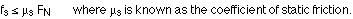The coefficient of friction (static or kinetic) is a measure of how difficult it is to slide a material of one kind over another; the coefficient of friction applies to a pair of materials, and not simply to one object by itself.

Note that there is a less-than-or-equal-to sign in the equation for the static frictional force. The static force of friction has a maximum value, but when two surfaces are not moving relative to each other the static force of friction is always just enough to exactly balance any forces trying to produce relative motion.

What happens when one object is sliding over another, when there is relative motion between two surfaces? There will still be a frictional force, but because we're dealing with things in motion we call it the kinetic frictional force. There is a different coefficient of friction associated with kinetic friction, the kinetic coefficient of friction, which is always less than or equal to the static coefficient.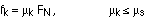As with the static frictional force, the kinetic frictional force acts to oppose the relative motion of the surfaces in contact. One important difference between the two is that the kinetic friction equation has an equals sign: the kinetic force of friction is always equal to the kinetic coefficient of friction times the normal force.

### Applying Newton's laws

Let's try a couple of examples involving friction.

Example 1 - A box of mass 3.60 kg travels at constant velocity down an inclined plane which is at an angle of 42.0° with respect to the horizontal. A string tied to the box exerts a vertical force of 7.38 N. What is the kinetic coefficient of friction?

Again, start by drawing a picture. In this case, because the box is traveling down the ramp, we know the frictional force is kinetic. It opposes the motion, so the frictional force must be directed up the slope. One thing to keep in mind in this problem is that the velocity is constant - this means the acceleration is zero.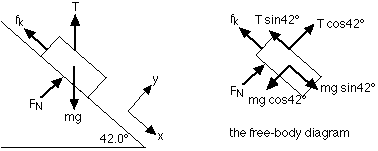The free-body diagram is also shown, with the forces split into components parallel and perpendicular to the inclined plane. Because there is no acceleration, any coordinate system is fine - a system parallel and perpendicular to the ramp is pretty convenient, though, because two of the forces are along those directions.

Applying Newton's second law to the forces in the y-direction :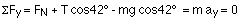Again, we can use this equation to solve for the normal force: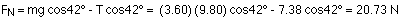Applying Newton's second law in the x-direction gives: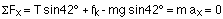The box is moving at a constant velocity, so that means the acceleration is zero. Solving for the kinetic force of friction gives: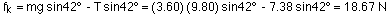The coefficient of kinetic friction can be found from the normal force and the frictional force: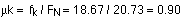This is actually a relatively large value for the coefficient, so it's not easy to move this box along this ramp.

### Example 2

Consider another example involving an inclined plane, only this time there will be two boxes involved. This is quite a challenging example, so don't be too intimidated if it looks tricky. Start by seeing if you agree with the free-body diagrams; if you understand those, you've made an important step in learning some physics.

Box 1, a wooden box, has a mass of 8.60 kg and a coefficient of kinetic friction with the inclined plane of 0.35. Box 2, a cardboard box, sits on top of box 1. It has a mass of 1.30 kg. The coefficient of kinetic friction between the two boxes is 0.45. The two boxes are linked by a rope which passes over a pulley at the top of the incline, as shown in the diagram. The inclined plane is at an angle of 38.0° with respect to the horizontal. What is the acceleration of each box?

The diagram for the situation looks like this: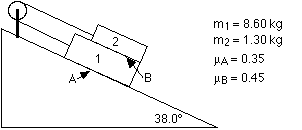The next step is to draw a free-body diagram of each box in turn. To draw these, it helps to think about which way the boxes will accelerate. The two boxes are tied together, and the heavier box will win...in other words, it will accelerate down the slope and the lighter one, on top, will be accelerated up towards the pulley. The pulley, by the way, simply changes the direction of the tension force. We're assuming that the pulley is massless and frictionless, so both boxes feel the same tension force. It's important to know the direction of the acceleration (or, if you don't know, to guess) and apply what you figured out (or your guess) consistently to both boxes.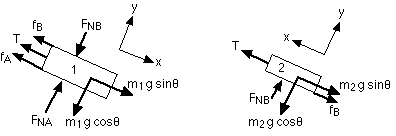The free-body diagram of box 1 is relatively complicated, with a total of 6 forces appearing. The free-body diagram for box 2 is a little easier to deal with, having 4 forces, so that's a good place to start.

For box 2 - start by summing the forces in the y-direction, where there is no acceleration:This can be solved to give the normal force: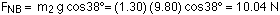Now find the net force in the x direction, where there is an acceleration up the slope:There are two unknowns in this equation, the tension T and ax, the acceleration. We can at least solve for T in terms of ax, like this:Now move on to box 1. Going back to the free-body diagram and summing forces in the y-direction gives:This equation can be solved to give the normal force associated with the interaction between the inclined plane and box 1: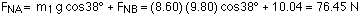Things are a little more complicated in the x-direction, but adding up the forces gives: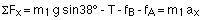substituting in the expression we worked out for T, and what fB and fA are gives: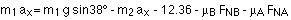Moving the acceleration terms to the left side gives: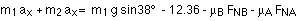Solving for the acceleration gives: